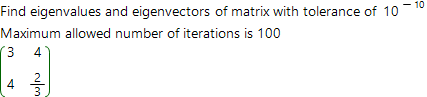Matrix eigenvalues and eigenvectors calculator

The number λ is called eigenvalue of the matrix A, if there is non-zero vector x such, that:

A x = λ x

This online calculator finds eigenvalues and eigenvectors of the matrix with step by step solution. To find the solution, calculator uses the numerical algorithm. To start working, this algorithm needs to be given desired accuracy for solution finding and number of iterations to spend.

Matrix eigenvalues and eigenvectors calculatorA =